Introduction to Analysis : Differentiability on Real Numbers (R)

Example Questions

Example Question #1 : Intro Analysis

Determine whether the following statement is true or false:

Let, and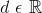. If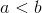and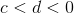then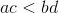.

True

False

False

Explanation:

Determine this statement is false by showing a contradiction when actual values are used.

Let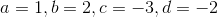First make sure the inequalities hold true.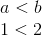and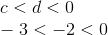Now find the products.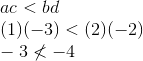Therefore, the statement is false.